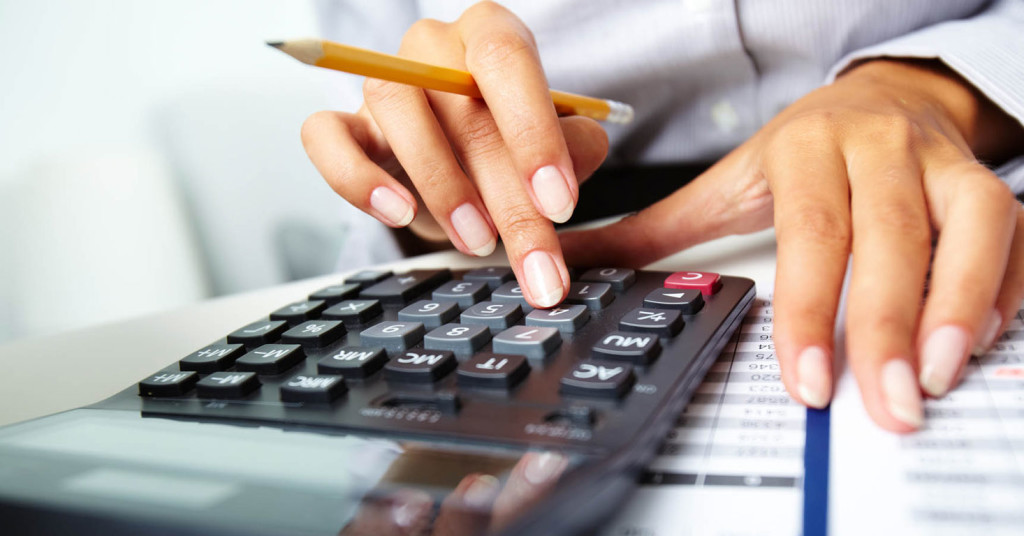# Fundamental Taxation Equation: Problems and Solutions

The accounting system is built by the accounting equation and it is the foundation of the system. The basic accounting equation is a base for the double entry system of accounting. The simple equation of accounting states two facts and it is all about a company. It talks about the company’s owes and owns.First, we have to understand the basics of the accounting equation because it is very important before entering into this subject. The company’s financial position is calculated by the following points:

• The Assets what the company owns like cash in hand, cash at the bank, bills receivable and short-term investments.
• The Liabilities what the company owes from others like the loan from a bank, bills payable, bad debts, bank overdraft and Sundry creditors.
• The Capital is the distinction between liabilities and assets.

The accounting equations denotes a relationship among the following manner,

Asset= Capital + Liabilities

Liabilities = Asset-Capital

Capital = Asset-Liabilities

### Here are few solutions for basic accounting equation:

a). Commenced business with Capital \$ 200,000

Solution

Capital (200,000) + Liabilities (0) = Assets (Cash =200,000)

b). Bought machinery \$20,000

Solution

Capital (200,000) + Liabilities (0) = Assets (Cash= 180,000 + Machinery= 20,000)

c). Bought goods for \$ 20,000
Solution

Capital (200,000) + Liabilities (0) = Assets (Cash=160,000+Machinery=20,000+Goods=20,000)

d). Brought goods from Mr. John on credit \$ 10,000

Solution

Capital (200,000) + Liabilities (John=10,000) = Asset (Cash=160,000+Machinery=20,000+Goods=30,000)

e). Sold goods for Cash \$25,000

Solution

Capital (200,000) + Liabilities (John=10,000) = Asset (Cash= 185,000+Machinery=20,000+Goods=5000)

f). Sold goods to Mr. Thomas on credit \$2000

Solution
Capital (200,000) + Liabilities (John 10,000) = Asset (Cash 185,000+Machinery=20,000+Goods=3000+Thomas=2000)

g). Paid cash to John (8000)

Solution

Capital (200,000) + Liabilities (John 2000) = Asset (Cash=177,000+Machinery=20000+Goods=3000+Thomas=2000)

h). Received cash from Thomas (1000)

Solution

Capital (200,000) + Liabilities (John 2000) = Asset (Cash= 178,000+Machinery=20,000+Goods=3000+Thomas=1000)

The solving method of accounting equation will differ according to the problem and there are many solving methods for it. A corporate and a sole proprietorship are having different equation in accounting equation. Though there are many easy methods to solve the problems, it consists many problems which we need to find a solution.

#### A Corporate:

Basic accounting equation for the Corporate,

Assets = Stockholder’s equity + Liabilities

#### A Sole Proprietorship:

This is a business which under the control of a person and he is the owner of this sole proprietorship.

#### The problems of fundamental taxation equation:

There are many problems in fundamental taxation equation. Corporate needs an accounting equation and it is the main problem. A sole proprietorship also needs accounting equation, and the equation helps it to rid out of the problems. The missing account must be calculated in the shareholder’s equity. When expanding the accounting equation for the sole proprietorship and also when expanding the accounting equation for the corporation. Whenever we do such equation of accounting, we will get many problems in it because it needs more concentration. When we start to look it clearly, we can understand the solving method easily without getting any mistake.

#### Solving the problems: Fundamental Taxation Equation: Problems and Solutions

The accounting system is built by the accounting equation and it is the foundation of the system. The basic accounting equation is a base for the double entry system of accounting. The simple equation of accounting states two facts and it is all about a company. It talks about the company’s owes and owns.